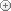Flume - Sintra lyrics correction | LyricsFreak.com

# Correct Sintra Lyrics

 Artist: Song: Comment: Lyrics:  Let me, know you Let me, know you Let me, know you Let me, know you (Here on the floor) Let me, know you (Here on the floor) Let me, know you here on the floor Let me, come on, know you here on the floor Let me, know you here on the floor Let me, know you (Here on the floor) Let me, know you here on the floor Let me, come on, know you here on the floor Let me, know you here on the flooradd rows